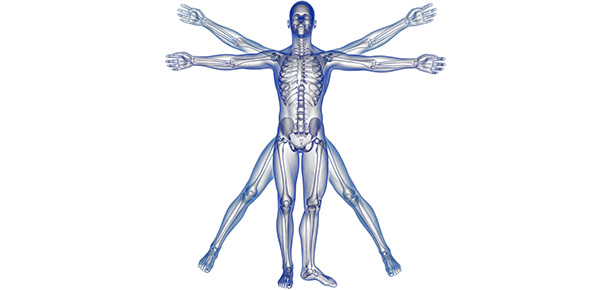# Amazing Human Body Facts

25 Questions | Attempts: 256SettingsBy Eddie Hung 2014

• 1.
How many bones in an adult human?
• A.

68

• B.

120

• C.

206

• D.

317

• 2.
Which is the hardest structure in the human body?
• A.

Enamel of the tooth

• B.

Middle of long bone

• C.

Pelvis

• D.

Ear bones

• 3.
Which is the smallest bone in the body?
• A.

Last tail bone

• B.

An ear bone called stapes

• C.

The bone inside the nose

• D.

The middle waist bone

• 4.
How many bones in the hand?
• A.

5

• B.

13

• C.

36

• D.

54

• 5.
How many times faster do the finger nails grow compared to that of toe nails?
• A.

2

• B.

4

• C.

6

• D.

8

• 6.
How many cells are there in a human body?
• A.

100 thousands

• B.

100 billions

• C.

100 trillions

• D.

1000 trillion

• 7.
How many cells are replaced every hour?
• A.

1000

• B.

10,000

• C.

One million

• D.

One billion

• 8.
How much Oxygen is required by an adult human daily?
• A.

900 gm

• B.

8 Kg

• C.

40 Kg

• D.

120 Kg

• 9.
What is the total length of all blood capillaries in a human body?
• A.

56 km

• B.

12,800 km (the diameter of the earth)

• C.

100,000 km (2.5 times of the circumference of the arth)

• D.

1 million km

• 10.
What is the total surface area of all the air sacs (inside the lungs) in a human body?
• A.

2.74 sq m (size of a table tennis table)

• B.

436 sq m (size of a basketball court)

• C.

18,000 sq m (size of a footy ground)

• D.

70 sq m (size of a tennis court)

• 11.
How much blood is filtered to form urine in a day?
• A.

24 litres

• B.

48 litres

• C.

180 litres

• D.

368 litres

• 12.
How many cells do each square metre of skin have?
• A.

5500

• B.

12,000

• C.

3.5 millions

• D.

6.8 millions

• 13.
What is the length of the small intestine?
• A.

3 m

• B.

6 m

• C.

10 m

• D.

12 m

• 14.
What is the total internal surface of the small intestine?
• A.

6 sq m

• B.

60 sq m

• C.

600 sq m

• D.

6000 sq m

• 15.
How many bacteria can be found in our large intestine in percentage of dry mass of faeces?
• A.

10%

• B.

25%

• C.

45%

• D.

60%

• 16.
What is the amount of saliva produced per day?
• A.

35 ml

• B.

500 ml

• C.

1.7 litres

• D.

2.9 litres

• 17.
How many hairs do a person have?
• A.

50,000

• B.

100,000

• C.

450,000

• D.

1 million

• 18.
Which organ performs the most number of functions?
• A.

Lung

• B.

Liver

• C.

Thyroid gland

• D.

Breast

• 19.
Which is the largest organ in the body?
• A.

Lung

• B.

Small intestine

• C.

Skin

• D.

Diaphragm

• 20.
How many facial muscles do you have?
• A.

13

• B.

25

• C.

42

• D.

53

• 21.
How many brain cells in a person?
• A.

1 billions

• B.

10 billions

• C.

100 billions

• D.

1 trillions

• 22.
How long will red blood cells live?
• A.

56 days

• B.

120 days

• C.

2 years

• D.

Forever

• 23.
How many heartbeats do a person have in a year ?
• A.

7200 times

• B.

150,000 times

• C.

1.5 millions

• D.

35 millions

• 24.
What is the percentage of the mass of the brain?
• A.

2%

• B.

12%

• C.

22%

• D.

32%

• 25.
How much Oxygen is used by the brain?
• A.

2%

• B.

11%

• C.

20%

• D.

32%

## Related TopicsBack to top
×

Wait!
Here's an interesting quiz for you.